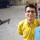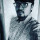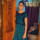1. Home >
2. Apps >
3. Groups >

# Difference between ac and dc analysis and transient analysis?

Question asked by Priyanka S G in #Electronics on Feb 25, 2013Priyanka S G · Feb 25, 2013
Rank E2 - BEGINNER

What is difference between ac analysis, dc analysis and transient analysis?

Posted in: #ElectronicsJeffrey Arulraj · Feb 26, 2013
Rank A2 - PRO
Consider an Hybrid circuit (Which uses both AC and DC supply) like an Common Emitter amplifier Here there are two inputs. One is the DC supply and the other is the AC input at the Base

To find the response of the system with only the DC supply and zero AC supply we do DC analysis.

To find the response of the amplifier to the AC input only we use AC analysis

And no circuit in practice gives the desired output instantly as supply is turned on. There is a time called settling time which charges all the dynamic components to the required value. This time taken to do these initial setting ups is called as Transient time and the analysis here is called transient timeAbhishek Rawal · Feb 26, 2013
Rank A2 - PRO
Priyanka S G
What is difference between ac analysis,dc analysis and transient analysis?
First of all,

What's Analysis ?
In any Network circuit, there are numerous capacitors,resistors,dependent & independent sources.
Now, using the basic concepts like KVL,KCL,initial condition,Millman's Theorem,Norton's Theorem,Superposition Theorem, etc. you are understanding each & every component of the network (like how much energy x capacitor stores at some specific time, how much voltage is obtained at Y node).This is called Analysis.

Now AC Analysis & DC Analysis are almost same with some changes.
Transient Analysis - I have no idea !

Let's make it an interesting/educating debate :
Conqueror
Consider an Hybrid circuit (Which uses both AC and DC supply) like an Common Emitter amplifier Here there are two inputs. One is the DC supply and the other is the AC input at the Base
Why an Amplifier uses AC & DC supply both when only AC supply is to be amplified ? 😉
(Just a debate)Jeffrey Arulraj · Feb 27, 2013
Rank A2 - PRO
Troll_So_Hard
First of all,

Let's make it an interesting/educating debate :

Why an Amplifier uses AC & DC supply both when only AC supply is to be amplified ? 😉
(Just a debate)
The most important thing we need to understand is this OUTPUT POWER CAN NEVER EXCEED input power.

This said let us consider the input AC power of an amplifier in some milli watts. The amplifier output is always in the range of Watts.

Here we find input power is way to less than the output AC power got from the amplifier. This difference in power is got from the DC source applied to the amplifier circuit.

SO now let us define an AMPLIFIER

It is a circuit which uses DC power from a DC source to magnify the AC input signal to the desired power ratingKarshil Sheth · Jan 26, 2020
Rank C2 - EXPERT

On DC analysis, every receptive segment are disregarded (they simply suggest a transient during start/stop) and capacitors are just open circuit and inductors a short circuit (or coil resistance), just resistance matters and is utilized for biassing.

Air conditioning examination requires aknowledging the reactances reactions at each recurrence as they might be intended for a particular range however may present motions on another upsetting the circuit behvior and, now and again, decimating it,Kavya Burramolla · Feb 1, 2020
Rank C1 - EXPERT

As per my understanding the circuit analysis is done to check and determine its charactersitics when it is imposed with different excitations. For example when AC supply is provided circuit characteristics are different from that f the circuit that is excited with the Dc supply.

Here to know the difference between AC analysis, DC analysis and transient analysis one must first know what are the basic characteristics that a circuit possess. VI(Voltage - Current) characteristics are the basic characteristics any circuit will have. It determines the behaviour of the circuit under different conditions.

Transient Analysis is carried out on the circuit to extract VI characteristics with respect to time. Ac analysis is carried out on the circuit to extract VI characteristics with respect to frequency. In short, Transient analysis gives time domain waveforms which are plots of voltage or current versus time. AC analysis gives the voltage or current versus frequency in a linearized version of the circuit. While DC analysis gives DC voltage or current, usually versus a stepped voltage or current.

## You must log-in or sign-up to reply to this post.

Click to Log-In or Sign-Up